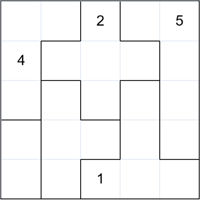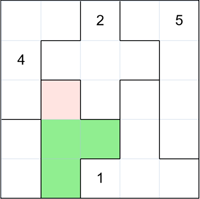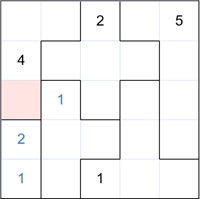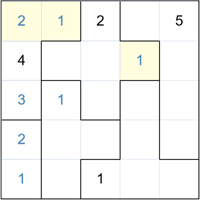## Suguru Basic WalkthroughOn the left is a 5x5 grid Suguru puzzle. The objective is to fill in all the empty cells such that each group of cells contains numbers 1 to the size of the group and no two adjacent cells - even diagonally - can contain the same number. So the block on the top left corner contains 4 cells and therefore will contain the numbers 1, 2, 3 and 4.
In this example we are given four cells already filled in to help us solve. Let's start.Consider the highlighted block of 4 cells. Note that the 3 green cells are adjacent to the number 1 and so cannot contain a 1. This means that there is only one cell left that can contain the 1 (highlighted in pink).Now consider the group of two cells in the bottom left. One must contain a 1 and one a 2. But the highlighted cell cannot contain a 1, so therefore it must be a 2.We have filled in a 1 in the second cell of that group.
Now consider the highlighted cell. That group still need a 1, 2 and 3. But the cell cannot contain a 1 or 2, therefore must be a 3.We can easily fill in the last two numbers in that group (shown in yellow). Also, you can see in the middle cross group, there is only one place left for a 1 (yellow cell).I think you get the idea by now. The rest is very easy to solve - here is the final puzzle completed.
All the puzzles of level "Easy" can be solved like this.